# JEE Main 2020 Physics Paper With Solutions Sept 3 (Shift 1)

Solving the questions of JEE Main 2020 Paper with Solutions Physics (Shift 1- 3rd Sept) will help the students to prepare efficiently for the exams. Practising these questions will help the students in increasing the problem-solving speed, understand the pattern of the question paper, and to self analyse the topics they have to focus more. Click on the link below to download the Physics 2020 shift 1 paper.

### September 3 Shift 1 - Physics

1. A block of mass m = 1 kg slides with velocity v = 6 m/s on a frictionless horizontal surface and collides with a uniform vertical rod and sticks to it as shown. The rod is pivoted about O and swings as a result of the collision making angle θ before momentarily coming to rest. If the rod has mass M = 2 kg, and length l = 1m, the value θ of is approximately: (take g = 10 m/s2)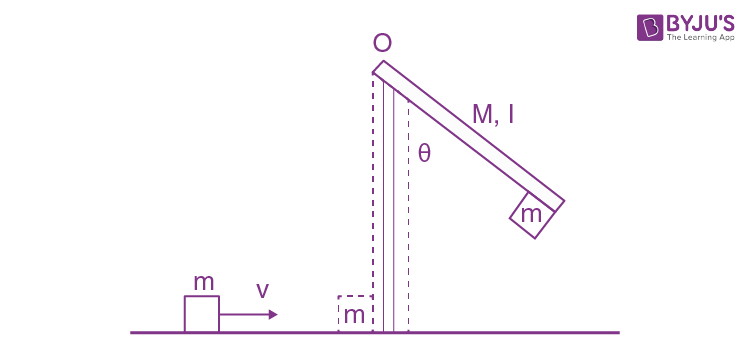1. 1) 49°
2. 2) 63°
3. 3) 69°
4. 4) 55°

Solution:

Applying law of conservation of momentum.

mvl = Iω

ω = mvl/I

From principle of conservation of energy.

$$\frac{1}{2}I\omega ^{2}=(m+M)gl_{com}(1-cos\theta )$$

$$\frac{1}{2}\frac{(mvl)^{2}}{I}=(m+M)gl_{com}(1-cos\theta )$$
-----------(1)

$$I=\left [ \frac{Ml^{2}}{3}+ml^{2} \right ]$$

$$I=\left [ \frac{2}{3}+1 \right ]=\frac{5}{3}$$

From equation, (1)

$$\frac{36\times 3}{2\times 5}=\frac{3\times 10\times 2}{3}(1-cos\theta )$$

$$\frac{27}{50}=(1-cos\theta )$$

$$cos\theta =1- \frac{27}{50}$$

$$cos\theta =\frac{23}{50}$$

$$\theta = 63^{0}$$

2. A uniform thin rope of length 12 m and mass 6 kg hangs vertically from a rigid support and a block of mass 2 kg is attached to its free end. A transverse short wave train of wavelength 6 cm is produced at the lower end of the rope. What is the wavelength of the wave train (in cm) when it reaches the top of the rope?

1. 1) 12
2. 2) 3
3. 3) 9
4. 4) 6

Solution:$$\mu =\frac{6}{12}=\frac{1}{2}kg/m$$

A→V=

$$\sqrt{\frac{T}{\mu }}=f\lambda$$

B→V’ =

$$\sqrt{\frac{T^{'}}{\mu }}=f\lambda^{'}$$

$$\sqrt{\frac{T}{T^{'}}}=\frac{\lambda }{\lambda ^{'}}$$

$$\sqrt{\frac{20}{80}}=\frac{6 }{\lambda ^{'}}$$

$$\lambda ^{'}=12$$

3. When a diode is forward biased, it has a voltage drop of 0.5 V. The safe limit of current through the diode is 10 mA. If a battery of emf 1.5 V is used in the circuit, the value of minimum resistance to be connected in series with the diode so that the current does not exceed the safe limit is:

1. 1) 300 Ω
2. 2) 200 Ω
3. 3) 50 Ω
4. 4) 100 Ω

Solution:1.5 = 1.5 + VR

⇒ VR = 1V

R = VR/I

= 1/ (10 x 10-3)

= 100 Ω

4. Using screw gauge of pitch 0.1 cm and 50 divisions on its circular scale, the thickness of an object is measured. It should correctly be recorded as:

1. 1) 2.125 cm
2. 2) 2.124 cm
3. 3) 2.123 cm
4. 4) 2.121 cm

Solution:

Least count =(0.1/50) =(1/500) = 0.002

when we multiply by division no.'s it must be even because L.C. is 0.002.

So, any measurement will be integral Multiple of LC.

So, ans. will be 2.124 cm

5. Model a torch battery of length l to be made up of a thin cylindrical bar of radius ‘a’ and a concentric thin cylindrical shell of radius ‘b’ filled in between with an electrolyte of resistivity ρ (see figure). If the battery is connected to a resistance of value R, the maximum joule heating in R will take place for: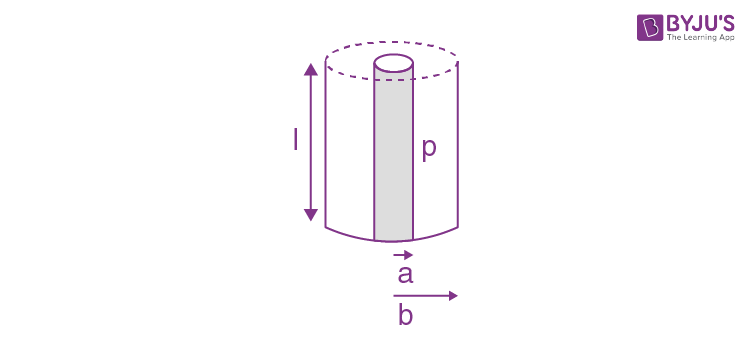1. 1)
$$R=\frac{\rho }{2\pi l}(\frac{b}{a})$$
2. 2)
$$R=\frac{2\rho }{\pi l}In(\frac{b}{a})$$
3. 3)
$$R=\frac{\rho }{\pi l}In(\frac{b}{a})$$
4. 4)
$$R=\frac{\rho }{2\pi l}In(\frac{b}{a})$$

Solution:

$$dR=\rho\frac{dr}{2\pi lr}$$

$$\int dR=\frac{\rho}{2\pi l}=\int_{a}^{b}\frac{\rho dr}{2\pi rl}ln\frac{b}{a}$$6. Consider a gas of triatomic molecules. The molecules are assumed to be triangular and made of massless rigid rods whose vertices are occupied by atoms. The internal energy of a mole of the gas at temperature T is:1. 1) (3/2)RT
2. 2) 3RT
3. 3) (5/2)RT
4. 4) (9/2)RT

Solution:

U =(f/2)nRT

U =(6/2)nRT

U = 3nRT

n = 1

U = 3RT

7. An elliptical loop having resistance R, of semi major axis a, and semi minor axis b is placed in magnetic field as shown in the figure. If the loop is rotated about the x-axis with angular frequency ω, the average power loss in the loop due to Joule heating is: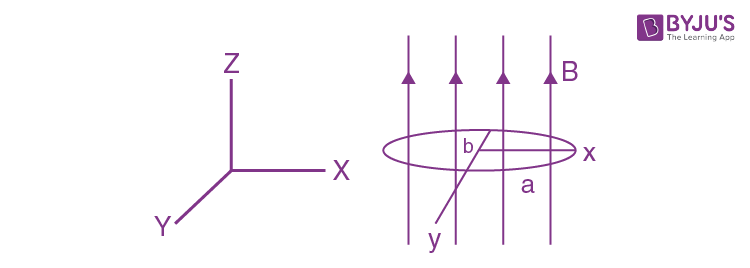1. 1) πabBω/R
2. 2) π2a2b2B2ω2/R
3. 3) π2a2b2B2ω2/2R
4. 4) Zero

Solution:

Φ =BA cos ωt

ε =(dφ/dt)= BA ω sin ωt

= Bπab ω sin ωt

ε0= Bπab ω

P = ε2/R = (π2a2b2B2ω2/R)(sin2 ωt) (Average value of sin2θ =1/2)

Pavg = π2a2b2B2ω2/2R

8. A balloon filled with helium (32° C and 1.7 atm.) bursts. Immediately afterwards the expansion of helium can be considered as:

1. 1) reversible isothermal
2. 2) irreversible isothermal

Solution:

irreversible adiabatic →Because Energy cannot be restored, if the process is sudden.

9. When the wavelength of radiation falling on a metal is changed from 500 nm to 200 nm, the maximum kinetic energy of the photoelectrons becomes three times larger. The work function of the metal is close to:

1. 1) 1.02 eV
2. 2) 0.61 eV
3. 3) 0.52 eV
4. 4) 0.81 eV

Solution:

$$KE_{max}=\frac{hc}{\lambda _{1}}-\phi$$

$$3KE_{max}=\frac{hc}{\lambda _{2}}-\phi$$

$$3\left ( \frac{hc}{\lambda _{1}}-\phi \right ) =\frac{hc}{\lambda _{2}}-\phi$$

$$\frac{3hc}{\lambda _{1}}-\frac{hc}{\lambda _{2}} =2\phi$$

$$\frac{3\times 1240}{500}-\frac{1240}{200} =2\phi$$

$$\phi = 0.62$$

10. Two isolated conducting spheres S1 and S2 of radius (2/3) R and (1/3) R have 12 μC and –3μC charges, respectively, and are at a large distance from each other. They are now connected by a conducting wire. A long time after this is done the charges on S1 and S2 are respectively:

1. 1) 6 μC and 3 μC
2. 2) 4.5 μC on both
3. 3) + 4.5 μC and –4.5 μC
4. 4) 3 μC and 6 μC

Solution: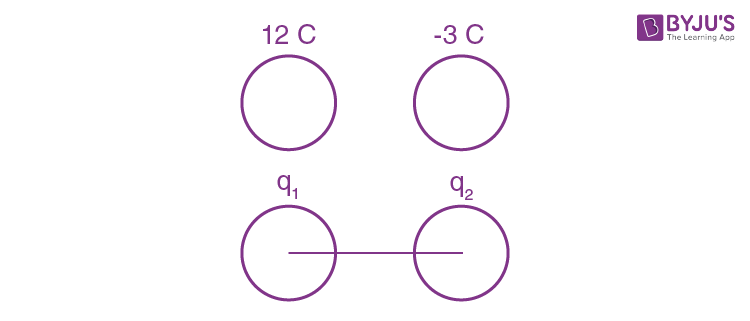q1+q2 = 9 μC------------------(1)

$$\frac{Kq_{1}}{\frac{2}{3}R}=\frac{Kq_{2}}{\frac{1}{3}R}$$

q1= 2q2

3 q2 =9 μC

q2 =3 μC

q1 =6 μC

11. In a radioactive material, fraction of active material remaining after time t is 9/16. The fraction that was remaining after t/2 is:

1. 1) 3/4
2. 2) 7/8
3. 3) 4/5
4. 4) 3/5

Solution:

N= N0 e-λt

$$\left [ \frac{N}{N_{0}} \right ]=e^{-\lambda t}$$

$$\left [ \frac{9}{16} \right ]=e^{-\lambda t}$$

After t/2 time

N/ N0= N0 e-λt/2

N/ N0= (9/16) ½ = ¾

12. Moment of inertia of a cylinder of mass M, length L and radius R about an axis passing through its centre and perpendicular to the axis of the cylinder is

$$I=M\left(\frac{R^{2}}{4}+\frac{L^{2}}{12}\right)$$
. If such a cylinder is to be made for a given mass of a material, the ratio L /R for it to have minimum possible I is:

1. 1) 2/3
2. 2) 3/2
3. 3) √2/3
4. 4) √3/2

Solution:

M =d.V

M=dπR2L

$$I=M\left(\frac{R^{2}}{4}+\frac{L^{2}}{12}\right)$$

$$I=M\left(\frac{M}{4 d \pi L}+\frac{L^{2}}{12}\right)$$

$$I=\left(\frac{M^{2}}{4 d \pi L}+\frac{ML^{2}}{12}\right)$$

$$\frac{dI}{dL}=\frac{M^{2}}{4 d \pi }\left ( \frac{-1}{L^{2}} \right )+\frac{2ML}{12}=0$$

$$\frac{M^{2}}{4 d \pi }\left ( \frac{1}{L^{2}} \right )=\frac{2ML}{12}$$

$$\frac{d\pi R^{2}L}{4 d \pi L^{2}}=\frac{L}{6}$$

$$\frac{R^{2}}{L^{2}}=\frac{2}{3}$$

$$\frac{R}{L}=\sqrt{\frac{2}{3}}\Rightarrow \frac{L}{R}=\sqrt{\frac{3}{2}}$$

13. A satellite is moving in a low nearly circular orbit around the earth. Its radius is roughly equal to that of the earth’s radius Re. By firing rockets attached to it, its speed is instantaneously increased in the direction of its motion so that it become √3/2 times larger. Due to this the farthest distance from the centre of the earth that the satellite reaches is R. Value of R is:

1. 1) 2Re
2. 2) 3Re
3. 3) 4Re
4. 4) 2.5 Re

Solution:

$$V_{0}=\sqrt{\frac{GM}{R_{e}}}\times \sqrt{\frac{3}{2}}$$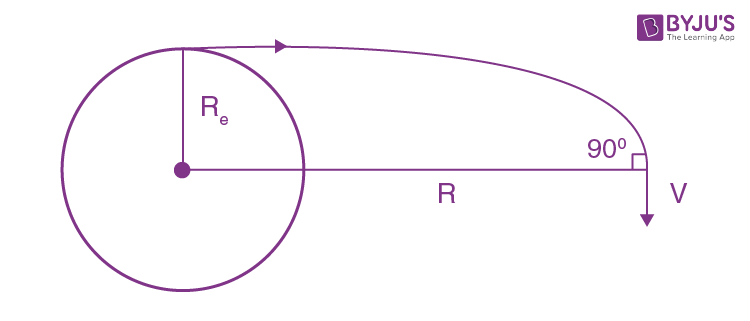From conservation of angular momentum about the centre of earth mV0 Re= mVR

$$\sqrt{\frac{GM}{R_{e}}}\times \sqrt{\frac{3}{2}}R_{e}=VR$$

From conservation of energy:

$$-\frac{GMm}{R_{e}}+ \frac{1}{2}mv_{0}^{2}= -\frac{GMm}{R}+ \frac{1}{2}mv^{2}$$

$$-\frac{GMm}{R_{e}}+ \frac{1}{2}m\frac{3}{2}\frac{GM}{R_{e}}= - \frac{GMm}{R}+ \frac{1}{2}m\frac{3}{2}\frac{GM}{R_{e}}\frac{R_{e}^{2}}{R^{2}}$$

$$-\frac{1}{R_{e}}+ \frac{3}{4R_{e}}= - \frac{1}{R}+ \frac{3}{4}\frac{R_{e}}{R^{2}}$$

$$-\frac{1}{4R_{e}}= - \frac{1}{R}+ \frac{3}{4}\frac{R_{e}}{R^{2}}$$

By further calculating

R = 3Re

14. Pressure inside two soap bubbles are 1.01 and 1.02 atmosphere, respectively. The ratio of their volumes is:

1. 1) 4 : 1
2. 2) 2 : 1
3. 3) 0.8 : 1
4. 4) 8 : 1

Solution:

$$P_{in}=P_{0}+\frac{4T}{R_{1}}$$

$$1.01=1+\frac{4T}{R_{1}}$$

$$0.01=\frac{4T}{R_{1}}$$

$$0.02=\frac{4T}{R_{2}}$$

$$\frac{1}{2}=\frac{R_{2}}{R_{1}}$$

$$\frac{V_{1}}{V_{2}}=\frac{8}{1}$$

15. In a Young’s double slit experiment, light of 500 nm is used to produce an interference pattern. When the distance between the slits is 0.05 mm, the angular width (in degree) of the fringes formed on the distance screen is close to:

1. 1) 0.17°
2. 2) 0.07°
3. 3) 0.57°
4. 4) 1.7°

Solution:

B = λD/d

Θ = β/D = (λ/d)

$$\theta =\frac{500 \times 10^{-9}}{0.05 \times 10^{-3}}$$

$$\theta =\frac{5 \times 10^{-2}}{5}$$

$$\theta^{0} =\frac{5}{100}\times \frac{180}{5\times \pi }$$

$$\theta^{0} =0.57^{0}$$

16. A 750 Hz, 20 V (rms) source is connected to a resistance of 100 Ω, an inductance of 0.1803 H and a capacitance of 10 μ F all in series. The time in which the resistance (heat capacity 2 J/°C) will get heated by 10°C. (assume no loss of heat to the surroundings) is close to:

1. 1) 245 s
2. 2) 365 s
3. 3) 418 s
4. 4) 348 s

Solution:

f = 750Hz,

Vrms = 20V,

R = 100 Ω,

L = 0.1803H

C = 10 μ f

S = 2J / C

$$z=\sqrt{R^{2}+\left(X_{L}-X_{c}\right)^{2}}=\sqrt{R^{2}+\left(2 \pi f L-\frac{1}{2 \pi f c}\right)^{2}}$$

$$\left |z \right |=\sqrt{100^{2}+\left(2\times 3.14\times 750\times 0.1803-\frac{1}{2\times 3.14\times 750\times 10^{-5}}\right)^{2}}$$

$$\left |z \right |= 834 \Omega$$

In AC,

P= VrmsIrms cosφ

$$P=\left [ V_{rms}.\frac{V_{rms}}{\left | Z \right |}.\frac{R}{\left | Z \right |} \right ]$$

$$P=\frac{V_{rms}}{\left | Z \right |}^{2}.R$$

$$P=\left (\frac{20}{834} \right )^{2}.100 = 0.0575J/S$$

P×t = S∆θ

t=2(10)/0.0575

t = 348 sec

17. Magnitude of magnetic field (in SI units) at the centre of a hexagonal shape coil of side 10 cm, 50 turns and carrying current I (Ampere) in units of μ0I/π is:

1. 1) 250√3
2. 2) 50√3
3. 3) 500√3
4. 4) 5√3

Solution: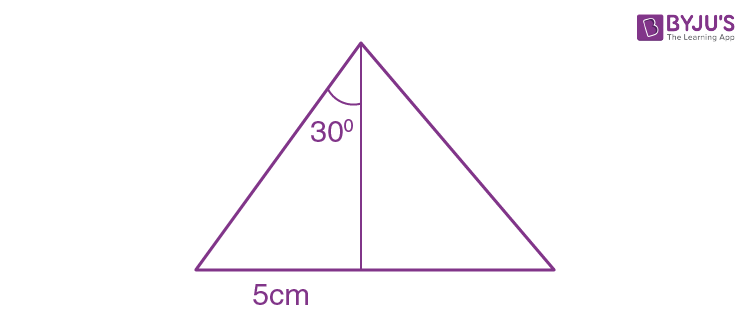tan 30 = x/d

d = x/tan 30

d = (5 x 10-2/{1/√3})

d = 5√3 x 10-2

$$\begin{array}{l} B=\frac{6 \times \mu_{0} I N}{4 \pi d}\left(\sin \theta_{1}+\sin \theta_{2}\right) \\ B=\frac{\mu_{0} I}{4 \pi} \times \frac{50 \times 6}{5 \sqrt{3} \times 10^{-2}}(\sin 30+\sin 30) \end{array}$$

$$B=\frac{\mu_{0} I}{\pi} \times \frac{10 \times 6\times 100}{\sqrt{3} \times 4}$$

B= 500√3

18. The magnetic field of a plane electromagnetic wave is

$$\vec{B}= 3 \times 10^{-8}sin\left [ 200\pi (y+ct) \right ]\hat{i}T$$

where c = 3 × 108 ms–1 is the speed of light.

The corresponding electric field is:

1. 1)
$$\vec{E}= -9sin\left [ 200\pi (y+ct) \right ]\hat{k}V/m$$
2. 2)
$$\vec{E}= 9sin\left [ 200\pi (y+ct) \right ]\hat{k}V/m$$
3. 3)
$$\vec{E}= -10^{-6}sin\left [ 200\pi (y+ct) \right ]\hat{k}V/m$$
4. 4)
$$\vec{E}= 3\times 10^{-8}sin\left [ 200\pi (y+ct) \right ]\hat{k}V/m$$

Solution:

If the wave is travelling along +y direction and its direction is along

$$\vec{E}\times \vec{B}$$

E = BC

E = 3 x 10-8 x 3 x 108

E = 9

$$\vec{S}=\frac{\vec{E}\times \vec{B}}{r}$$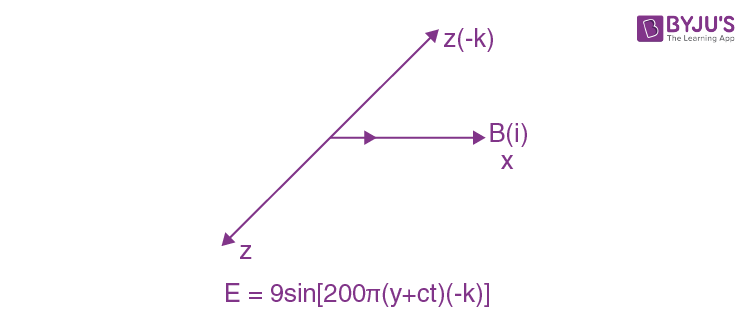19. A charged particle carrying charge 1 μC is moving with velocity

$$(2\hat{i}+3\hat{j}+4\hat{k})ms^{-1}$$
. If an external magnetic field of
$$(5\hat{i}+3\hat{j}-6\hat{k})\times 10^{-3}T$$
exists in the region where the particle is moving then the force on the particle is
$$\vec{F}\times 10^{-9}N$$
. The vector
$$\vec{F}$$
is :

1. 1)
$$(-0.30\hat{i}+0.32\hat{j}-0.09\hat{k})$$
2. 2)
$$(-3.0\hat{i}+3.2\hat{j}-0.9\hat{k})$$
3. 3)
$$(-30\hat{i}+32\hat{j}-9\hat{k})$$
4. 4)
$$(-300\hat{i}+320\hat{j}-90\hat{k})$$

Solution:

$$\overrightarrow{\mathrm{F}}=q(\overrightarrow{\mathrm{V}} \times \overrightarrow{\mathrm{B}})$$

$$\overrightarrow{\mathrm{F}}=10^{-6}(2 \hat{\mathrm{i}}+3 \hat{\mathrm{j}}+4 \hat{\mathrm{k}}) \times(5 \hat{\mathrm{i}}+3 \hat{\mathrm{j}}-6 \hat{\mathrm{k}}) \times 10^{-3} \\$$

$$=10^{-6}\left|\begin{array}{ccc} \mathrm{i} & \hat{\mathrm{j}} & \hat{\mathrm{k}} \\ 2 & 3 & 4 \\ 5 & 3 & -6 \end{array}\right|$$

$$=10^{-6}[\hat{\mathrm{i}}(-18-12)-\hat{j}(-12-20)+\hat{\mathrm{k}}(6-15)]\\$$

$$\overrightarrow{\mathrm{F}}=(-30 \hat{\mathrm{i}}+32 \hat{\mathrm{j}}-9 \hat{\mathrm{k}}) \times 10^{-9}$$

20. In the circuit shown in the figure, the total charge is 750 μC and the voltage across capacitor C2 is 20 V. Then the charge on capacitor C2 is: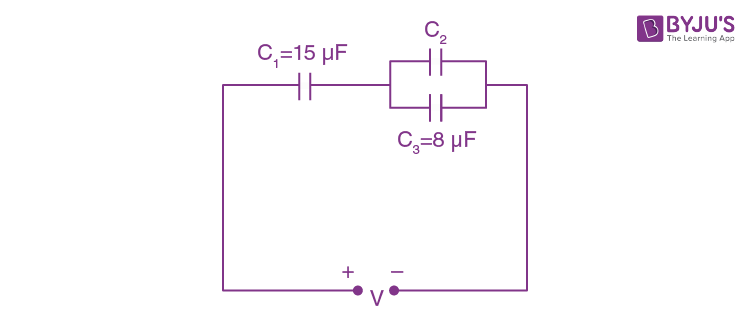1. 1) 650 μC
2. 2) 450 μC
3. 3) 590 μC
4. 4) 160 μC

Solution: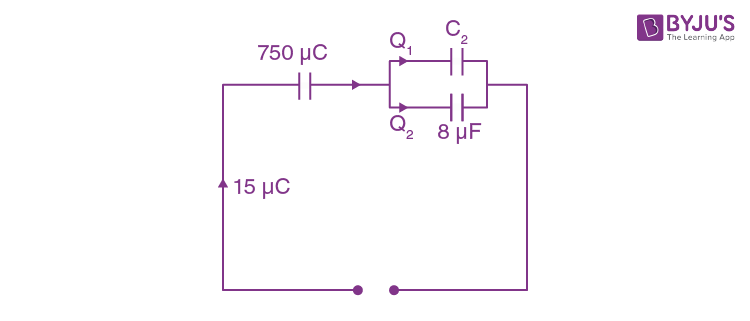Potential difference across C2 & C3 are equal

Q2= CV = 8 X 20 = 160 μC

Q1= 750 – 160 = 590 μC

21. A person of 80 kg mass is standing on the rim of a circular platform of mass 200 kg rotating about its axis at 5 revolutions per minute (rpm). The person now starts moving towards the centre of the platform. What will be the rotational speed (in rpm) of the platform when the person reaches its centre _____?

Solution:

I1ω1 = I2ω2

$$\left(\frac{M R^{2}}{2}+m R^{2}\right) \omega_{1}=\omega_{2} \frac{M R^{2}}{2}$$

$$\left(1+\frac{2 m R^{2}}{M R^{2}}\right) \omega_{1}=\omega_{2}$$

$$\left(1+\frac{2 \times 80}{200}\right) \omega_{1}=\omega_{2}$$

ω2 = ω1 1.8

2π f2=2 πf1 x1.8

f2=5 x1.8= 9

22. An observer can see through a small hole on the side of a jar (radius 15 cm) at a point at height of 15 cm from the bottom (see figure). The hole is at a height of 45 cm. When the jar is filled with a liquid up to a height of 30 cm the same observer can see the edge at the bottom of the jar. If the refractive index of the liquid is N/100, where N is an integer, the value of N is _____.

Solution:

1.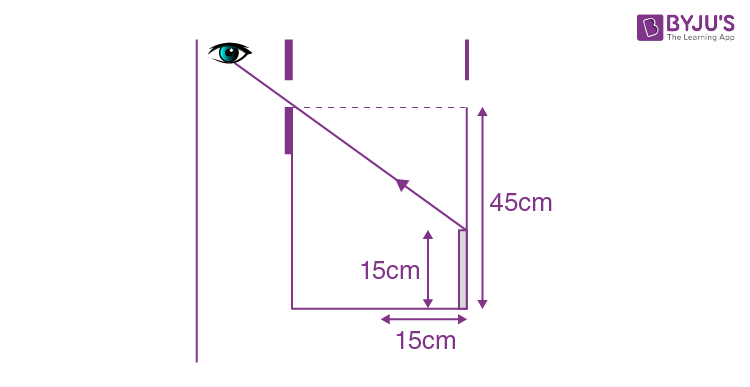2.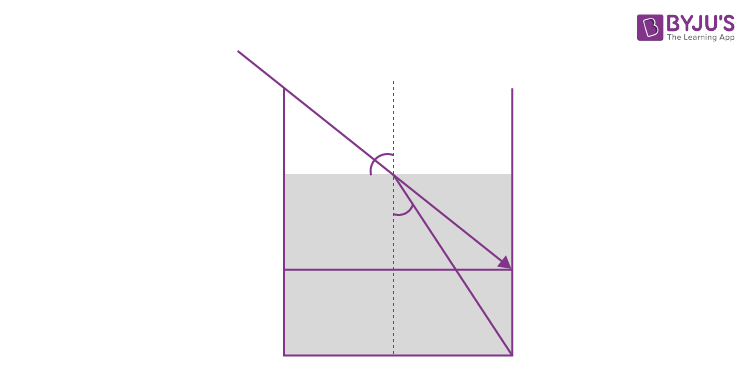$$\mu sini=1sin45$$

$$\mu \frac{15}{\sqrt{1125}}=\frac{1}{\sqrt{2}}$$

$$\mu =\frac{\sqrt{1125}}{15\sqrt{2}}$$

$$\mu =\frac{\sqrt{1125}}{\sqrt{450}}$$

μ=1.58

μ = N/100 = 1.58

N = 158

23. A cricket ball of mass 0.15 kg is thrown vertically up by a bowling machine so that it rises to a maximum height of 20 m after leaving the machine. If the part pushing the ball applies a constant force F on the ball and moves horizotally a distance of 0.2 m while launching the ball, the value of F(in N) is (g = 10 ms–2)_____.

Solution:

1.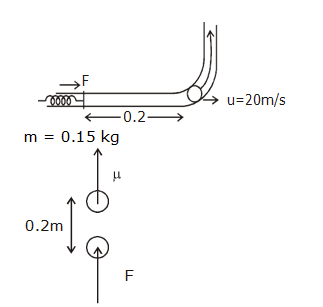F x 0.2 = mgh

F x 0.2 = 0.15 x 10 x 20

F = (3/2)x [(10 x20)/2]

F = 150 N

24. When a long glass capillary tube of radius 0.015 cm is dipped in a liquid, the liquid rises to a height of 15 cm within it. If the contact angle between the liquid and glass to close to 0°, the surface tension of the liquid, in milliNewton m–1, is [ρ(liquid) =900 kgm–3 , g = 10 ms–2] (Give answer in closes integer)____.

Solution:

r = 0.015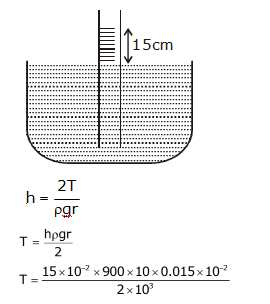T = 101

25. A bakelite beaker has volume capacity of 500 cc at 30°C. When it is partially filled with Vm volume (at 30°C) of mercury, it is found that the unfilled volume of the beaker remains constant as temperature is varied. If ϒ(beaker)= 6 × 10–6 °C–1 and ϒ (mercury)= 1.5 × 10–4 °C–1, where ϒ is the coefficient of volume expansion, then Vm (in cc) is close to ____.

Solution:

For the volume to remain constant at all temperature

Exp. of the liquid = Exp. of solid

∆V = vϒ∆T

V1ϒ1= V2ϒ2

500cc × 6 × 10-6 = Vm ×1.5×10-4

Vm = (500 x 6 x 10-6)/(1.5 x 10-4) = 30/1.5

Vm = 20 cc

### JEE Main 2020 Physics Paper With Solutions Shift 1 September 3